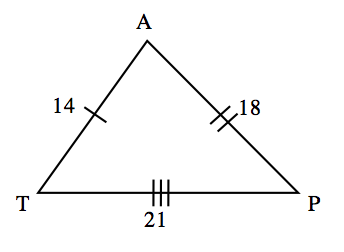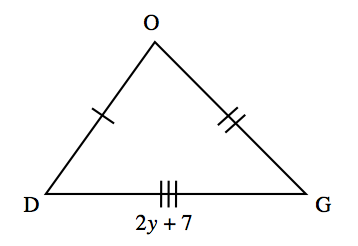### Home > CCG > Chapter 7 > Lesson 7.2.5 > Problem7-96

7-96.

Suppose you know that $ΔTAP≅ΔDOG$ and that $TA=14$, $AP=18$, $TP=21$, and $DG=2y+7$.

1. On your paper, draw a reasonable sketch of $ΔTAP$ and $ΔDOG$.2. Find $y$. Show all work.

How are these triangles related? How can that relationship help you find $y$?

$2y+7=21$, because corresponding sides are congruent (equal).